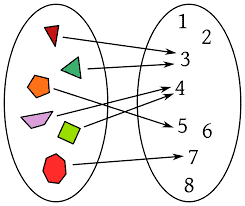Foundations of Mathematics
Problem 1.

Prove that if $a$ and $b$ are odd integers, then $4 \chi\left(a^{2}+b^{2}\right)$.

Proof .

Problem 2. Prove that if $x$ is an integer, then $x^{3}$ has the same parity as $x$.

Proof .

Problem 3. (a) Let $n \in \mathbb{Z}$. Prove that if $2 \mid\left(n^{2}-5\right)$, then $4 \mid\left(n^{2}-5\right)$.
(b) Give an example of an integer $n$ such that $2 \mid\left(n^{2}-5\right)$, but $8 \chi\left(n^{2}-5\right)$

Proof .

Problem 4.

Consider the statement:
“If the product of two integers is even, then at least one of these integers is even.”
(a) Rewrite the statement in symbols.
(b) Give a formal proof.

Proof .

Problem 5. Let $a$ be a positive real number. Prove that there is a unique positive real number $x$ such that $x^{4}-a^{4}=0 .$

Proof .

Problem 6. Prove by induction that if $n$ is a positive integer, then $9^{n}-4^{n} \in 5 \mathbb{Z}$.

Proof .

$9^{n+1}-4^{n+1}=5 \in 5\times 9^{n}+4(9^{n}-4^{n})$

CATALOG DESCRIPTION: See catalog description here

Math 300 is designed to provide a bridge between computational mathematics and theoretical mathematics (“real math”). Thus a major goal is to teach the students how to write proofs.

The required core of topics include logic, set theory, number theory, induction, functions, relations, operations, and combinatorics. Since there is an emphasis on how to create and write proofs, most professors find that these topics are sufficient for the semester. If time permits, the professor may choose to teach other topics, such as graph theory.

TO THE INSTRUCTOR: The main trouble spot is that students attempt to learn proofs in the same way that they learned concepts in calculus. Thus considerable time must be spent showing students how to connect given information to what it is they are trying to prove with logical deductions. Considerable time should be spent in office hours coaching students through proofs that they have tried on their own.

Math 300 is a w-course (writing intensive) for our department. Our majors need two such courses in order to graduate. The requirements for a w-course are:

1. 1/3 of the grade for math 300 should be determined by the writing component of the course.
2. At least 2000 words (eight pages) of graded, finished writing are assigned. Note: In addition to assigned papers this may include exams, quizzes, and homework.
3. Includes some writing instruction.
4. Provides formative feedback on writing in progress. If a paper is assigned, then a rough draft should be critiqued and returned to the student, with only the final version being graded.
5. Distributes assignments so as to allow feedback before a final draft is due and students have some indication by mid-term of the quality of their writing, even if on drafts.
6. Students must pass the writing portion of the course to pass.
7. Does not allow undergraduate aides to grade more than ten percent of the writing portion of the final grade (with the exception of Calibrated Peer Review software).

For more details see the following web site: Faculty ResourcesMATH 300 – Foundations of Mathematics代写认准UpriviateTA

BS equation代写